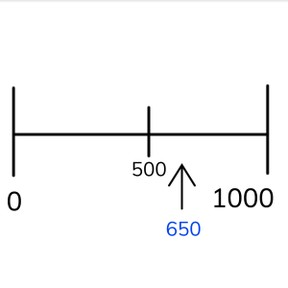Approximate placement of numbers to 1,000 on the number line

# Approximate placement of numbers to 1,000 on the number line

Approximate placement of numbers to 1,000 on the number line8,000 schools use Gynzy92,000 teachers use Gynzy1,600,000 students use Gynzy

## General

Students learn to approximately place numbers to 1,000 on the number line, on a blank number line only showing 0 and 1,000.

2.NBT.A.2

## Relevance

Discuss with students that it is important to be able to approximately place numbers on the number line. If you know where a number approximately belongs, it helps you to determine which number is bigger or smaller, because you know which numbers a given number is close to.

## Introduction

The number line shown goes from 0 to 1,000. Have students draw a line from the given numbers to the place they belong on the number line. Then have students skip count in 100s from a random number on the number line.

## Development

Show students a number line without marks and one with marks. Ask students what the differences are. Discuss that on the first number line you can place a number exactly where it belongs, whereas on the second number line you approximate the placement. Discuss the term "approximate" and explain that it means that you are not exactly sure, but have a good idea of something. Tell students that when they approximate placement of a number on a blank number line, that they should start by finding the middle. The middle of 0 and 1,000 is 500. Once you've found the middle, decide if the number is bigger or smaller than the middle. Determine the neighboring hundreds numbers of the number and then place your number. In this example it is between 100 and 200. The number is closer to 200. The second number is 910. Explain that you can use another method to find approximate placement. First, find the middle of 0 and 1,000. That is 500. Then find the middle of 0 to 500 (250) and 500 to 1,000 (750). The number 910 is between 750 and 1,000 which shows you where your number approximately belongs. Next students must choose from given answers, and then approximately place numbers. Finally they must indicate which number they think belongs at a given arrow.

Check that students understand approximate placement of numbers to 1,000 by asking the following questions:
- Which steps can help to approximately place a number?
- Which numbers are helpful to place first on a blank number line to 1,000?
- How do you approximately place a number on the number line?

## Guided practice

Students practice approximate placement of numbers on an empty number line, where the middle is indicated. Ask students how they came to decide where to place the number and to name the steps they took. Summarize the steps by indicating that the number was closer to 500 than 1,000. The answer is 650, so a number between 600 and 700 is good. Next students can choose from multiple choice answers to answer which number has been approximately placed. The question mark is closer to 1,000 than 500 but not so close that 800 or 900 are possible answers. Students must determine which approximate amount is reasonable. Tell them that it is close to 500. Have students write down a range in which the number could fall and discuss this together.

## Closing

Discuss with students that approximately placing numbers to 1,000 on the number line helps to determine which numbers are larger and smaller. Check that students know how to approximately place a number on the number line in the correct steps. First by finding the middle (500) and then determining if the number is bigger or smaller. They then can choose to find neighboring hundreds numbers or find the middle of 0 to 500 (250) and 500 to 1,000 (750) and determing approximately where to place the number. Have students determine which numbers belong at the arrows on the interactive whiteboard. They can choose from four options.

## Teaching tips

Let students who have difficulty with approximate placement of numbers to 1,000 on the number line make a number line to 1,000 and use that during the calculations. Help them practice the steps to take to determine approximate location. You can use number cards during this process.

## Instruction materials

Number cards to 1,000; tens and hundreds

### The online teaching platform for interactive whiteboards and displays in schools

• Save time building lessons

• Manage the classroom more efficiently

• Increase student engagement